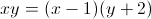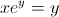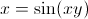# How to Find Derivatives of Implicit Functions - Quiz & Worksheet

Instructions:

question 1 of 3

### Find y'.Create Your Account To Take This Quiz

As a member, you'll also get unlimited access to over 84,000 lessons in math, English, science, history, and more. Plus, get practice tests, quizzes, and personalized coaching to help you succeed.

Try it risk-free for 30 days. Cancel anytime

### 1. Find y'(x).### 2. Find y'(x).Create your account to access this entire worksheet
Quizzes, practice exams & worksheets
Certificate of Completion
Create an account to get started

Finding derivatives of implicit functions is an involved mathematical calculation, and this quiz and worksheet will allow you to test your understanding of performing these calculations. These practice questions will ask you to define the value of variables in implicit functions.

## Quiz & Worksheet Goals

In these assessments, you'll be tested specifically on your ability to define derivatives in practice problems that involve implicit functions. You will be provided with several different implicit functions and be asked to select the correct derivative from a list of options.

## Skills Practiced

• Reading comprehension - ensure that you draw the most important information about derivatives in implicit functions from the related lesson
• Problem solving - use your acquired knowledge to solve practice problems involving derivatives in implicit functions
• Knowledge application - use your knowledge to answer questions which involve knowing how to set up these functions in such a way that makes them solvable

Get more information through reading the lesson called How to Find Derivatives of Implicit Functions. The information in this lesson will help you further understand the following objectives:

• Define derivatives in implicit functions
• Understand the steps involved in implicit differentiation
• Go through example problems that ask you to find the derivative in an implicit function
Final ExamMath 104: Calculus
Status: Not Started
Chapter ExamCalculating Derivatives and Derivative Rules
Status: Not Started

Support